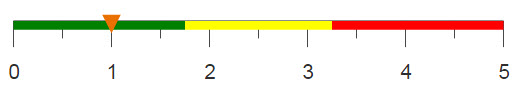# Selecting based on rank

You can select values based on certain criteria, for example if their rank puts them in a certain range.

## Difficulty## Objective

You want to know which ten stores sold the most of a specific product during a given time period. You know how to do this in SQL by using commands and functions such as SUM, WHERE, and GROUP BY, and you would like to know how to obtain the same results with 1010data.

## SQL solution

To find the ten stores that had the highest sales of item 406444 during 2015, you would execute the following query.

```SELECT store,sku,ROUND(SUM(qty)) AS `Sum of Sales`
FROM sales_item_detail
WHERE sku=406444 AND YEAR(date)=2015
GROUP BY store
ORDER BY SUM(qty) DESC
LIMIT 10;```

Using SELECT you can select the store number, SKU, and the sum of sales from the Sales Item Detail table. Additionally, you can select only the rows that contain the specified SKU and that occurred during the given time period. Grouping by the store and ordering by the sum of sales, you can obtain a list of the stores and their sales, ranked by the highest sum of sales. Using LIMIT, only the top ten stores are shown in the result.

With 1010data, these same results can be produced and additionally the results can be easily saved to a table or used in future queries. To use these results for additional analysis in SQL, you would have to nest this select statement within another query.

## 1010data Macro Language solution

```<base table="pub.doc.retail.altseg.sales_detail_transid"/>
<sel value="year(trans_date)=2015&sku=406444"/>
<tabu label="Sum of Product Sales" breaks="store,sku">
<tcol source="xsales" fun="sum" name="sum_xsales"
label="Sum of`Extended`Sales"/>
</tabu>
<sel value="g_rank(;;;sum_xsales)<=10"/>
<sort col="sum_xsales" dir="down"/>```

This analogue finds the ten stores that had the highest sales of the product with SKU 406444 during the year 2015. Therefore, you first use a selection statement to obtain only the transactions made in 2015 for SKU 4016444. A tabulation is then done to obtain the sum of sales for each store.

After the sum for each store is calculated, you can use the g_rank(G;S;O;X) to select the top ten stores with the highest sales. The <sort> operation is then used to mimic the results produced by SQL and order the stores by highest to lowest sales.

## Alternate 1010data Macro Language solution

```<base table="pub.doc.retail.altseg.sales_detail_transid"/>
<sel value="year(trans_date)=2015&sku=406444"/>
<tabu label="Sum of Product Sales" breaks="store,sku">
<tcol source="xsales" fun="sum" label="Sum of`Extended`Sales"/>
</tabu>
<sort col="t0" dir="down"/>
<sel value="i_<11"/>```

Alternatively, you can sort the Sum of Extended Sales column in descending order after the tabulation, and then use a selection statement to manually select the top ten stores.

## Further reading

If you would like to learn more about the functions and operations discussed in this recipe, click on the links below:

g_rank(G;S;O;X)

<tabu>